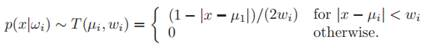# Consider a mixture distribution of two triangle distributions, where component density ωi is centered on µi and has “halfwidth” wi, according to: (a) Assume P(ω1) = P(ω2)=0.5 and derive the equations for the maximum likelihood values ˆµi and ˆwi, i = 1, 2. (b) Under the conditions in part (a), is the distribution identifiable? (c) Assume that both widths wi are known, but the centers are not. Assume, too, that there exist values for the centers that give non-zero probability to each of the samples. Derive a formula for the maximum-likelihood value of the centers. (d) Under the conditions in part (c), is the distribution identifiable?

Question

Consider a mixture distribution of two triangle distributions, where component density ωi is centered on µi and has “halfwidth” wi, according to:(a) Assume P(ω1) = P(ω2)=0.5 and derive the equations for the maximum likelihood values ˆµi and ˆwi, i = 1, 2.

(b) Under the conditions in part (a), is the distribution identifiable?

(c) Assume that both widths wi are known, but the centers are not. Assume, too, that there exist values for the centers that give non-zero probability to each of the samples. Derive a formula for the maximum-likelihood value of the centers.

(d) Under the conditions in part (c), is the distribution identifiable?